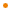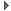# JavaFX: Bringing Rich Experiences To All the Screens Of Your Life

### Overview

The `Affine` class represents an affine transform. An affine transform performs a linear mapping from 2D coordinates to other 2D coordinates while preserving the "straightness" and "parallelness" of lines. Affine transformations can be constructed using sequences translations, scales, and shears.

Note: application developers should not normally use this class directly, but instead use the specific `Translate`, `Scale`, `Rotate`, or `Shear` transforms instead.

Such a coordinate transformation can be represented by a 3 row by 3 column matrix with an implied last row of [ 0 0 1 ]. This matrix transforms source coordinates `(x,y)` into destination coordinates `(x',y')` by considering them to be a column vector and multiplying the coordinate vector by the matrix according to the following process:

```	[ x']   [  m00  m01  m02  ] [ x ]   [ m00x + m01y + m02 ]
[ y'] = [  m10  m11  m12  ] [ y ] = [ m10x + m11y + m12 ]
[ 1 ]   [   0    0    1   ] [ 1 ]   [         1         ]
```

Profile: common

This comment needs review.

### Variable Summary

accessnametypedescription
publicm00Number1.0

Defines the X coordinate scaling element of the 3x3 matrix.

publicm01Number0.0

Defines the X coordinate shearing element of the 3x3 matrix.

publicm02Number0.0

Defines the X coordinate translation element of the 3x3 matrix.

publicm10Number0.0

Defines the Y coordinate shearing element of the 3x3 matrix.

publicm11Number1.0

Defines the Y coordinate scaling element of the 3x3 matrix.

publicm12Number0.0

Defines the Y coordinate translation element of the 3x3 matrix.

### Inherited Variables

#### javafx.scene.transform.Transform

accessnametypedescription

### Function Summary

public impl_getAffineTransform() : java.awt.geom.AffineTransformReturns
AffineTransform
&nbsp;

### Inherited Functions

#### javafx.scene.transform.Transform

public affine(m00: Number, m01: Number, m02: Number, m10: Number, m11: Number, m12: Number) : Affine

Returns a new `Affine` object from 6 number values representing the 6 specifiable entries of the 3x3 transformation matrix.Returns a new `Affine` object from 6 number values representing the 6 specifiable entries of the 3x3 transformation matrix.

Parameters
m00
the X coordinate scaling element of the 3x3 matrix
m01
the Y coordinate shearing element of the 3x3 matrix
m02
the X coordinate shearing element of the 3x3 matrix
m10
the Y coordinate scaling element of the 3x3 matrix
m11
the X coordinate translation element of the 3x3 matrix
m12
the Y coordinate translation element of the 3x3 matrix
Returns
Affine
a new `Affine` object derived from specified parameters

Profile: common

&nbsp;
public abstract impl_getAffineTransform() : java.awt.geom.AffineTransform

Constructs an `java.awt.geom.AffineTransform` object which performs the actual transformationConstructs an `java.awt.geom.AffineTransform` object which performs the actual transformation

Returns
AffineTransform
an `java.awt.geom.AffineTransform` object which performs the actual transformation
&nbsp;
public rotate(angle: Number, pivotX: Number, pivotY: Number) : Rotate

Returns a `Rotate` object that rotates coordinates around an anchor point.Returns a `Rotate` object that rotates coordinates around an anchor point. This operation is equivalent to translating the coordinates so that the anchor point is at the origin (S1), then rotating them about the new origin (S2), and finally translating so that the intermediate origin is restored to the coordinates of the original anchor point (S3).

The matrix representing the returned transform is:

```          [   cos(theta)    -sin(theta)    x-x*cos+y*sin  ]
[   sin(theta)     cos(theta)    y-x*sin-y*cos  ]
[       0              0               1        ]
```
Rotating by a positive angle theta rotates points on the positive X axis toward the positive Y axis.

Parameters
angle
the angle of rotation measured in degrees
pivotX
the X coordinate of the rotation anchor point
pivotY
the Y coordinate of the rotation anchor point
Returns
Rotate
a `Rotate` object that rotates coordinates around the specified point by the specified angle of rotation.

Profile: common

&nbsp;
public scale(x: Number, y: Number) : Scale

Returns a `Scale` object representing a scaling transformation.Returns a `Scale` object representing a scaling transformation.

The matrix representing the returned transform is:

```          [   sx   0    0   ]
[   0    sy   0   ]
[   0    0    1   ]
```

Parameters
x
the factor by which coordinates are scaled along the X axis direction
y
the factor by which coordinates are scaled along the Y axis direction
Returns
Scale
an <code>AffineTransform</code> object that scales coordinates by the specified factors.

Profile: common

&nbsp;
public scale(x: Number, y: Number, pivotX: Number, pivotY: Number) : Scale

Returns a `Scale` object representing a scaling transformation.Returns a `Scale` object representing a scaling transformation. The returned scale operation will be about the given pivot point.

Parameters
x
the factor by which coordinates are scaled along the X axis direction
y
the factor by which coordinates are scaled along the Y axis direction
pivotX
Defines the X coordinate about which point the scale occurs.
pivotY
Defines the Y coordinate about which point the scale occurs.
Returns
Scale
an <code>AffineTransform</code> object that scales coordinates by the specified factors.

Profile: common

&nbsp;
public shear(x: Number, y: Number) : Shear

Returns a `Shear` object representing a shearing transformation.Returns a `Shear` object representing a shearing transformation.

The matrix representing the returned transform is:

```          [   1   shx   0   ]
[  shy   1    0   ]
[   0    0    1   ]
```

Parameters
x
the multiplier by which coordinates are shifted in the direction of the positive X axis as a factor of their Y coordinate
y
the multiplier by which coordinates are shifted in the direction of the positive Y axis as a factor of their X coordinate
Returns
Shear
an <code>AffineTransform</code> object that shears coordinates by the specified multipliers.

Profile: common

&nbsp;
public translate(x: Number, y: Number) : Translate

Returns a `Translate` object representing a translation transformation.Returns a `Translate` object representing a translation transformation.

The matrix representing the returned transform is:

```          [   1    0    tx  ]
[   0    1    ty  ]
[   0    0    1   ]
```

Parameters
x
the distance by which coordinates are translated in the X axis direction
y
the distance by which coordinates are translated in the Y axis direction
Returns
Translate
a `Translate` object that represents a translation transformation, created with the specified vector.

Profile: common

&nbsp;

Spec-Zone.ru - all specs in one place